Geometry

# Properties of Circles Warmup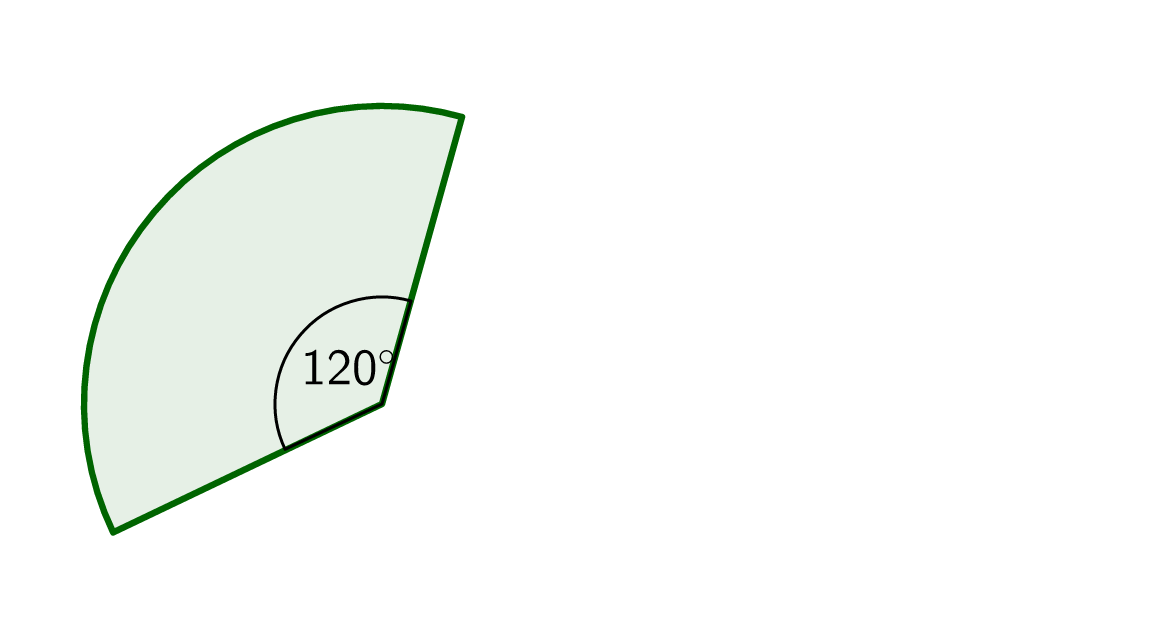The paper fan shown was cut from a circle whose area is $$18\pi.$$ What is the area of the fan?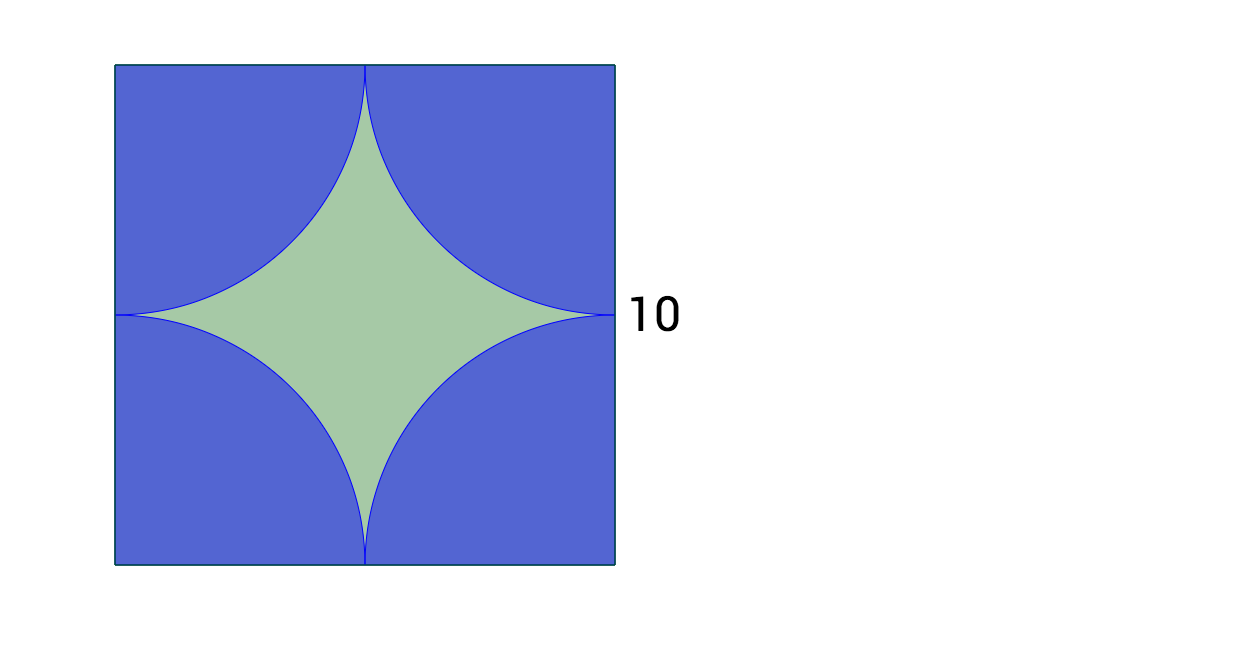In the square above, what is the area of the section shaded green?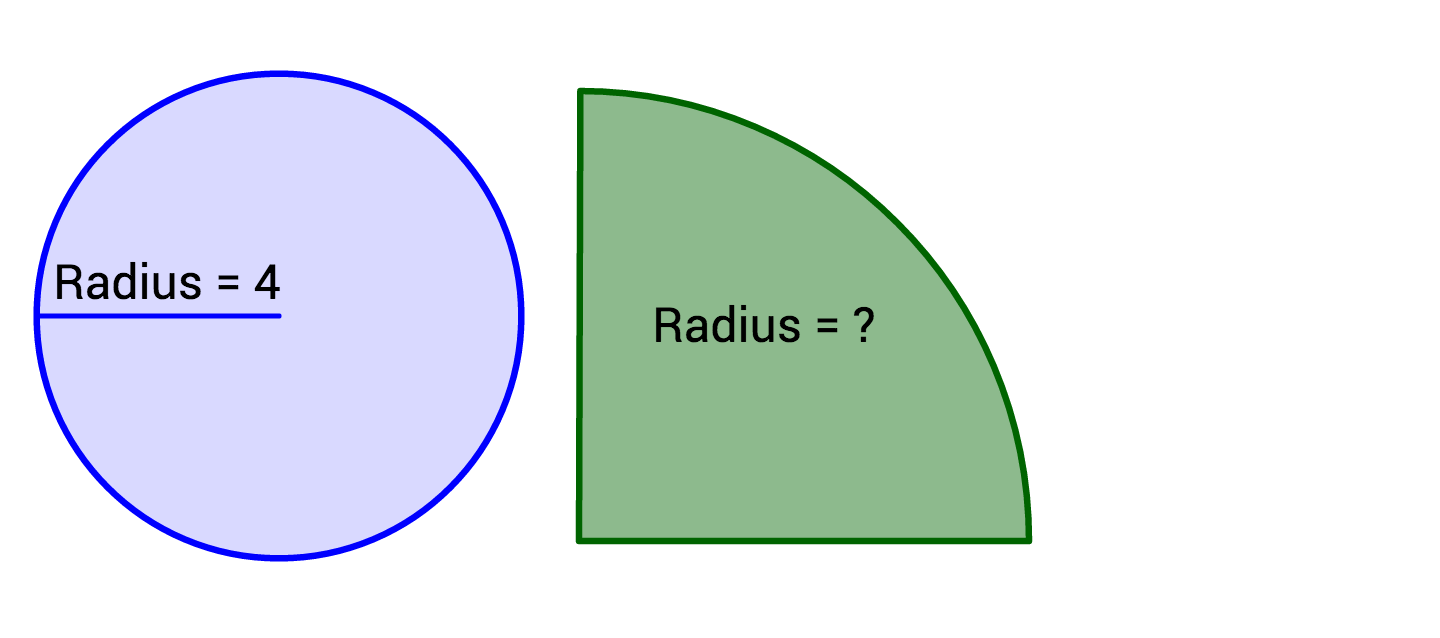A quarter circle has the same area as a circle with a radius of 4. What is the radius of the quarter circle?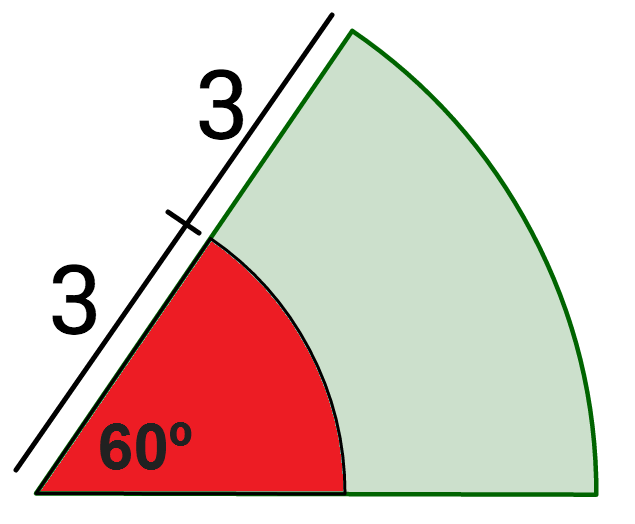What percent of the sector is shaded red?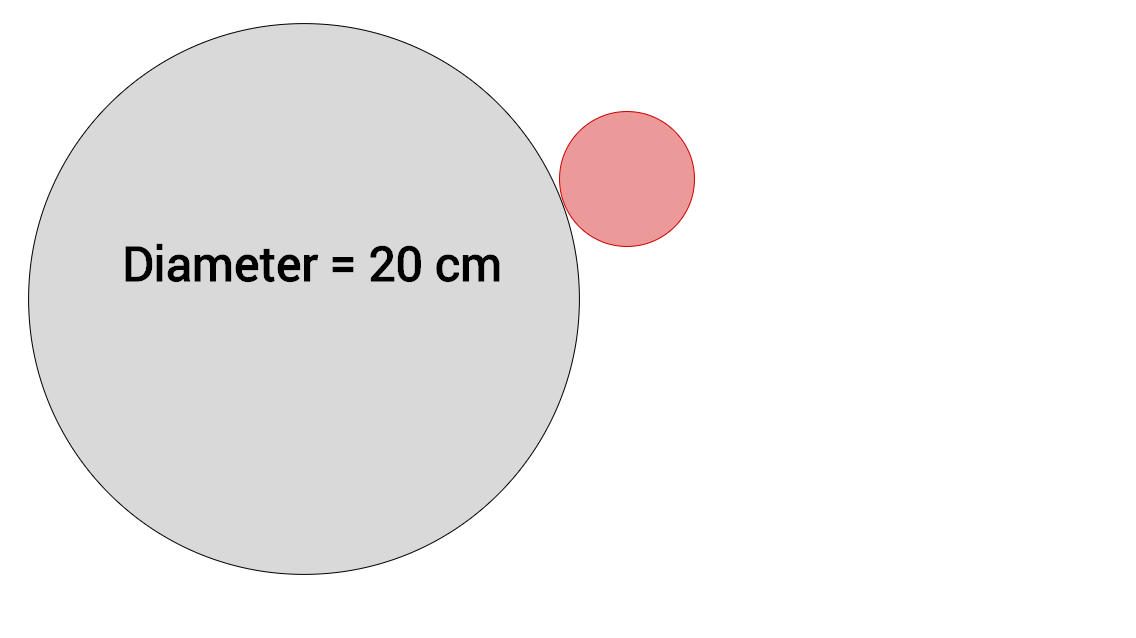A small circle rolls around the edge of a larger circle exactly 11 times to return to its starting location. If the large circle has a diameter of 20, what is the radius of the smaller red circle?

×